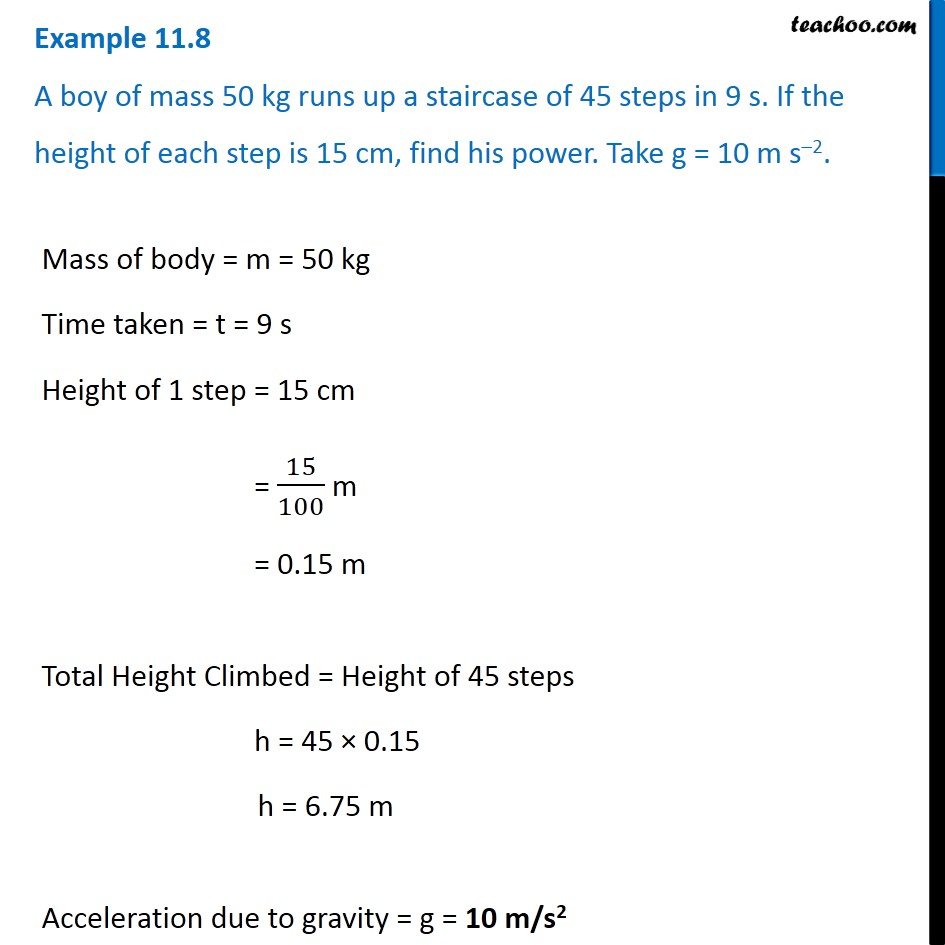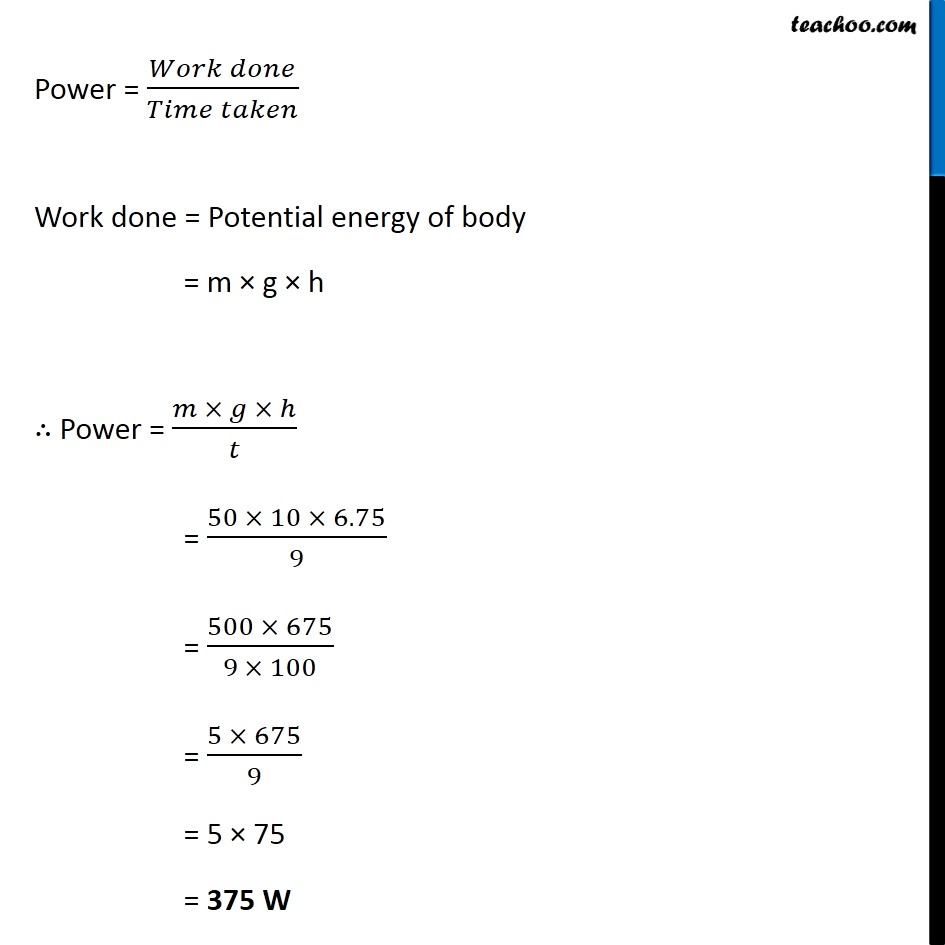Examples from NCERT Book

Class 9
Chapter 11 Class 9 - Work and EnergyLearn in your speed, with individual attention - Teachoo Maths 1-on-1 Class

### Transcript

Example 11.8 A boy of mass 50 kg runs up a staircase of 45 steps in 9 s. If the height of each step is 15 cm, find his power. Take g = 10 m s–2.Mass of body = m = 50 kg Time taken = t = 9 s Height of 1 step = 15 cm = 15/100 m = 0.15 m Total Height Climbed = Height of 45 steps h = 45 × 0.15 h = 6.75 m Acceleration due to gravity = g = 10 m/s2 Power = (𝑊𝑜𝑟𝑘 𝑑𝑜𝑛𝑒)/(𝑇𝑖𝑚𝑒 𝑡𝑎𝑘𝑒𝑛) Work done = Potential energy of body = m × g × h ∴ Power = (𝑚 × 𝑔 × ℎ)/𝑡 = (50 × 10 × 6.75)/9 = (500 × 675)/(9 × 100) = (5 × 675)/9 = 5 × 75 = 375 W